• 时间序列移动平均_时间序列-移动平均
2020-09-22 11:39:21时间序列移动平均

# 时间序列-移动平均 (Time Series - Moving Average)

For a stationary time series, a moving average model sees the value of a variable at time ‘t’ as a linear function of residual errors from ‘q’ time steps preceding it. The residual error is calculated by comparing the value at the time ‘t’ to moving average of the values preceding.

对于固定的时间序列，移动平均模型将时间“ t”处的变量值视为来自其前“ q”个时间步长的残差线性函数。 通过将时间“ t”处的值与先前值的移动平均值进行比较，可以计算出残余误差。

Mathematically it can be written as −

数学上可以写成-

$$y_{t} = c\:+\:\epsilon_{t}\:+\:\theta_{1}\:\epsilon_{t-1}\:+\:\theta_{2}\:\epsilon_{t-2}\:+\:...+:\theta_{q}\:\epsilon_{t-q}\:$$

$$y_ {t} = c \：+ \：\ epsilon_ {t} \：+ \：\ theta_ {1} \：\ epsilon_ {t-1} \：+ \：\ theta_ {2} \：\ epsilon_ {t-2} \：+ \：... +：\ theta_ {q} \：\ epsilon_ {tq} \：$$

Where‘q’ is the moving-average trend parameter

其中“ q”是移动平均趋势参数

$\epsilon_{t}$ is white noise, and

$\ epsilon_ {t}$是白噪声，并且

$\epsilon_{t-1}, \epsilon_{t-2}...\epsilon_{t-q}$ are the error terms at previous time periods.

$\ epsilon_ {t-1}，\ epsilon_ {t-2} ... \ epsilon_ {tq}$是先前时间段的误差项。

Value of ‘q’ can be calibrated using various methods. One way of finding the apt value of ‘q’ is plotting the partial auto-correlation plot.

可以使用多种方法来校准“ q”的值。 找到“ q”的合适值的一种方法是绘制局部自相关图。

A partial auto-correlation plot shows the relation of a variable with itself at prior time steps with indirect correlations removed, unlike auto-correlation plot which shows direct as well as indirect correlations, let’s see how it looks like for ‘temperature’ variable of our data.

部分自相关图显示了变量在先前时间步长上与自身之间的关系，其中间接相关性已删除，这与自相关图显示了直接相关性和间接相关性不同，让我们来看一下“温度”变量的样子数据。

## 显示PACP (Showing PACP)

In :

在中：


from statsmodels.graphics.tsaplots import plot_pacf

plot_pacf(train, lags = 100)
plt.show()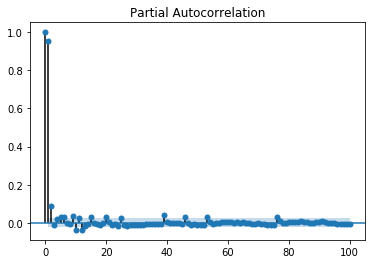A partial auto-correlation is read in the same way as a correlogram.

以与相关图相同的方式读取部分自相关。

时间序列移动平均python 机器学习 人工智能 java 算法
更多相关内容
• 基于次简单移动平均时间序列预测算法，取值可随机修改
• 移动窗口填补时间序列缺失值（imputeTS的matlab版本），包括简单移动平均值的简单缺失值插补； 线性加权移动平均值的线性缺失值插补； 指数加权移动平均值的指数缺失值插补
• 一般的移动平均法主要用于平稳序列，其主要思想就是通过近期几个（例如五个）的数据平均作为下一个即这五个之后的那个数据的预测值。 二次移动平均法的话就是在一次平均移动法的基础上一次平均移动计算出来的...

移动平均法
一般的移动平均法主要用于平稳序列，其主要思想就是通过对近期几个（例如五个）的数据求平均作为下一个即这五个之后的那个数据的预测值。

二次移动平均法的话就是在一次平均移动法的基础上对一次平均移动计算出来的值再进行一次移动平均。
思路还是十分简单明了的，只是公式看起来就有点乱七八糟了。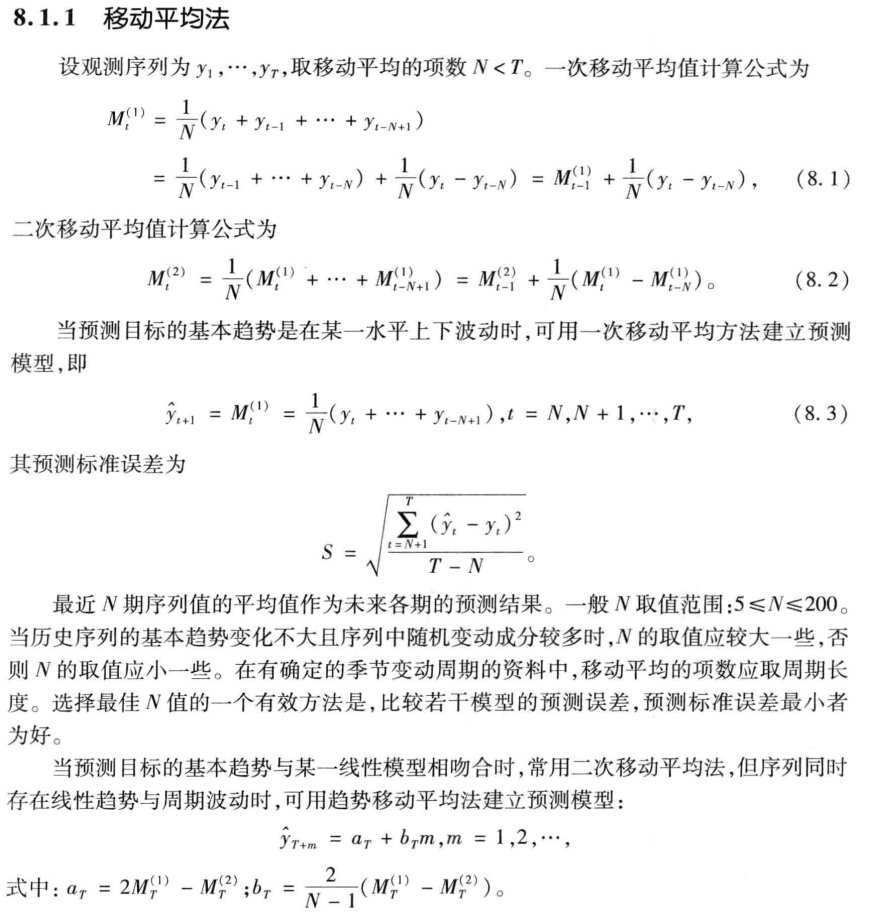下面展示一道例题：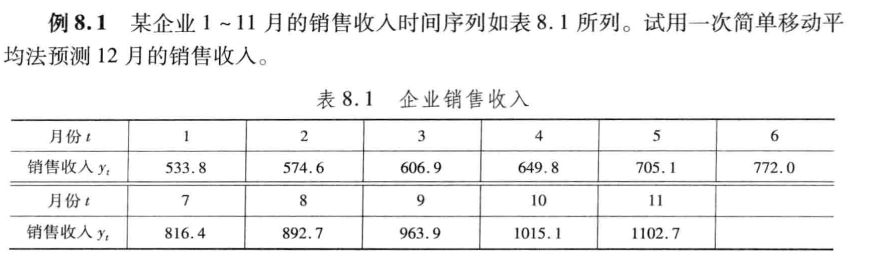clc,clear
y = [533.8 574.6 606.9 649.8 705.1 772.0 816.4 892.7 963.9 1015.1 1102.7];
m = length(y);
n = [4, 5]  % n为移动平均的项数，4就是4个求平均预测
for i = 1:length(n) % 由于n的取值不同，因此使用细胞数组
for j = 1:m - n(i) + 1
yhat{i}(j) = sum(y(j: j+n(i)-1))/n(i); % 从头到尾都自回归预测了一遍，最后一个才是我们需要的数
end
y12 = yhat{i}(end); % 取出需要12月的预测值
s(i) = sqrt(mean((y(n(i) + 1 : end) - yhat{i}(1:end - 1)).^2)); % 计算误差
end
y12 = yhat{1}(end)
y12 = yhat{2}(end)
s


分析：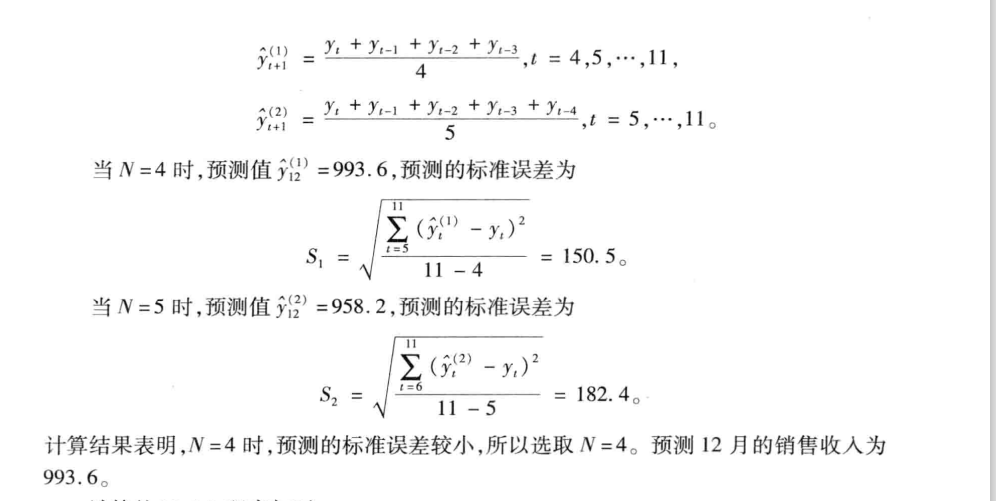本文参考的是司守奎，孙兆亮主编的数学建模算法与应用（第二版）

展开全文matlab
• 今天给大家讲解一下移动平均，其在时间序列分析中具有重要的作用。1 简介移动平均（moving average）主要应用于时间序列的分析，其能够去除不同时间步长的序列间的微小差异。移动平...

今天给大家讲解一下移动平均，其在时间序列分析中具有重要的作用。

## 1 简介

移动平均（moving average）主要应用于时间序列的分析，其能够去除不同时间步长的序列间的微小差异。

移动平均的目的是去除噪声

移动平均需要指定一个窗口大小（window size），称为窗口宽度（window width）。这定义了用于计算移动平均值的原始观测值的数量。

移动是指由窗宽定义的窗口沿时间序列滑动，计算新序列的平均值。

移动平均主要有两种形式：居中和截尾移动平均（Centered and Trailing Moving Average）。

居中移动平均

t 时刻的值计算由原始观测值在 t 时刻、之前和之后的平均值。

假设窗口为3，则：

center_ma(t) = mean(obs(t-1),obs(t),obs(t+1))

这个方法需要用到未来值。可以作为一种从时间序列中去除趋势和季节成分的通用方法，而在预测时通常不能使用这种方法。

截尾移动平均

t 时刻的值计算由原始观测值在 t 时刻以及之前的平均值。

假设窗口为3，则：

trail_ma(t) = mean(obs(t-2),obs(t-1),obs(t))

这个方法只用到历史数据，并可以用于时间序列预测。

本文主要侧重于截尾移动平均。

在应用移动平均时，需要对数据提出一些假设。假设趋势和季节成分已经从时序中去除。意味着数据是平稳的（stationary）或者未显示出明显的趋势（长期增长或下降）和季节性（一致的周期性结构）。

在时序数据预测时，有很多方法可以去除趋势和季节性，主要有差分法（differencing method）和建立行为模型，并显式地从序列中减去它。

接下来从三个面讲解截尾平均移动的应用。

## 2 移动平均的应用

### 2.1 数据准备

数据集是总共有365条记录，数据记录的是1959年加州每日出生的女婴数量。

链接：https://pan.baidu.com/s/1SpDi0oT-6jqKmAT4JwC0TA

提取码：6ylx

数据：daily-total-female-births.csv

代码：moving_average.py

显示数据的前5条记录：

Date,Births
1959/1/1,35
1959/1/2,32
1959/1/3,30
1959/1/4,31
1959/1/5,44


移动平均可以作为一种数据准备技术来创建原始数据集的平滑版本。

平滑（smoothing）是一种有用的数据准备技术，因为它可以减少观测中的随机变化。

pandas 中的 rolling() 函数可以自动根据窗口大小对观测数据进行移动平均。

假设窗口大小为3，则 t 时刻的转换值等于前三个观测值（t-2,t-1,t）的均值。

trans_obs(t) = 1/3 * ( obs(t-2) + obs(t-1) + obs(t) )

现在开始正式分析：

# 引入相关的包
import pandas as pd  # 表格和数据操作
import matplotlib.pyplot as plt
import numpy as np
from sklearn.metrics import mean_squared_error

# -------------------------------------------------------------------------------------
# 读入数据
birth.info()
plt.figure(figsize=(15, 7))
plt.plot(birth)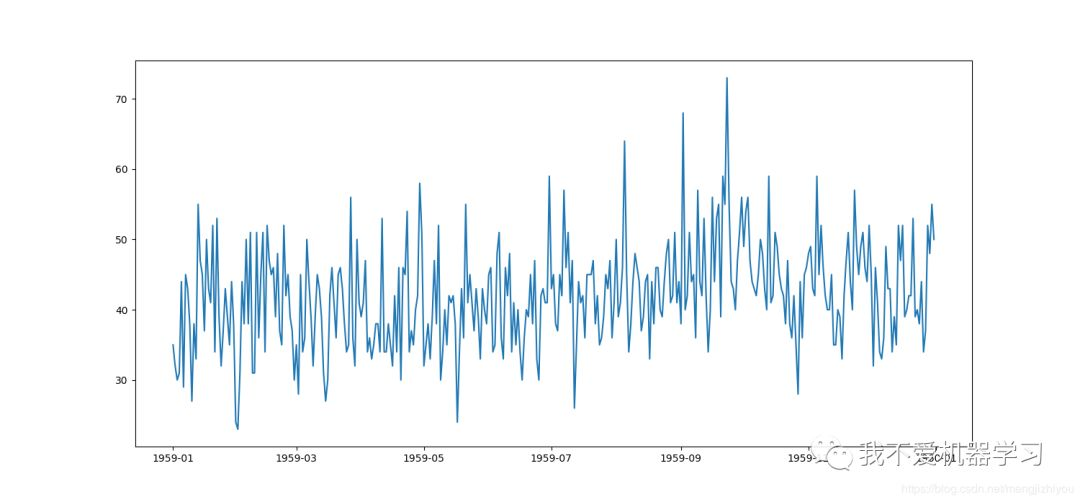从上图可以看出，这个数据集是研究移动平均方法的一个很好的例子，因为它没有显示任何明显的趋势或季节性。

下面是在窗口大小为3 的情况下的移动平均值。

window = 3
# trail-rolling average transform
rolling = birth.rolling(window=window)
rolling_mean = rolling.mean()
plt.figure(figsize=(15, 7))
plt.plot(birth)
plt.plot(rolling_mean, 'r')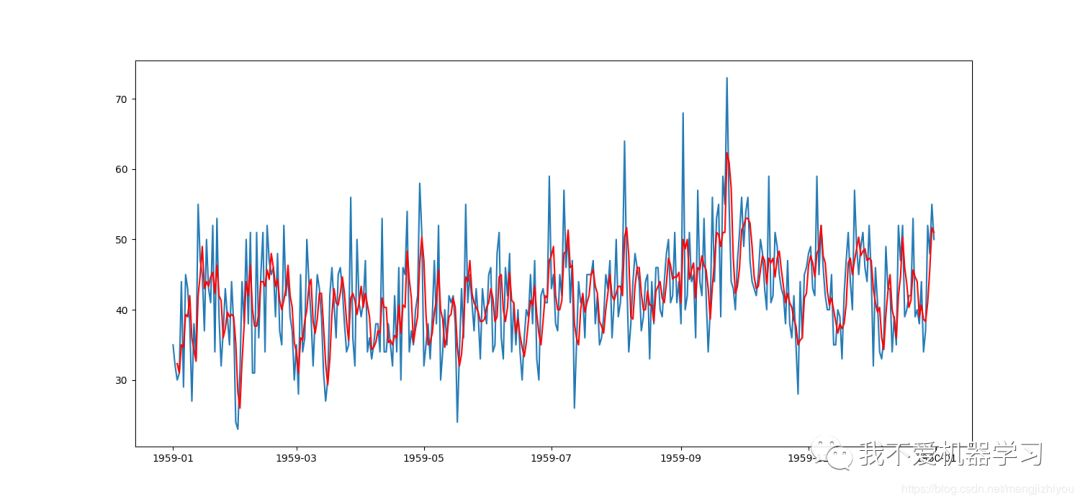从图中可以看出，原始观测值（蓝色）被移动平均转换值（红色）覆盖。

rolling_mean.head()

               Births
Date
1959-01-01        NaN
1959-01-02        NaN
1959-01-03  32.333333
1959-01-04  31.000000
1959-01-05  35.000000


移动平均值的前两个观测值是 NaN，因为前两个观测值的时间点在移动时缺少前面时刻的数据。这两个转换值需被删除。

### 2.2 特征工程

当建立时序预测模型可以被看做为监督学习问题时，移动平均可以作为新信息的来源。

在本例中移动平均计算得到的值可以作为一个新的特征。

lag1 = birth.shift(1)
lag3 = birth.shift(3)
lag3_mean = lag3.rolling(window=window).mean()
df = pd.concat([lag3_mean, lag1, lag3], axis=1)
df.columns = ['lag3_mean', 't-1', 't-3']



这里的 df 相当于是特征，本例中有三个特征，当把时序预测模型看做是监督问题时，此时的 Y 就是原始观测值 (Births)：

                  lag3_mean   t-1   t-3  Births
Date
1959-01-01        NaN   NaN   NaN      35
1959-01-02        NaN  35.0   NaN      32
1959-01-03        NaN  32.0   NaN      30
1959-01-04        NaN  30.0  35.0      31
1959-01-05        NaN  31.0  32.0      44
1959-01-06  32.333333  44.0  30.0      29


在建模时，将有NaN的行删除后再建模。

### 2.3 预测

移动平均值可以直接看做是预测值。

这是一个最基础的模型，其假设时间序列的趋势和季节性成分已经被删除或调整。

移动平均作为预测模型是直接向前走的形式，当有新的观测值，则这个模型会更新并预测。

# prepare situation
X = birth.values
history = [X[i] for i in range(window)]
test = [X[i] for i in range(window, len(X))]
predictions = []

# walk forward over time steps in test
for t in range(len(test)):
length = len(history)
yhat = np.mean([history[i] for i in range(length - window, length)])
predictions.append(yhat)
history.append(test[t])
print('predicted=%f, excepted=%f' % (yhat, test[t]))

error = mean_squared_error(test, predictions)
print('Test MSE: %3f' % error)
plt.figure(figsize=(15, 7))
plt.plot(test)
plt.plot(predictions, 'r')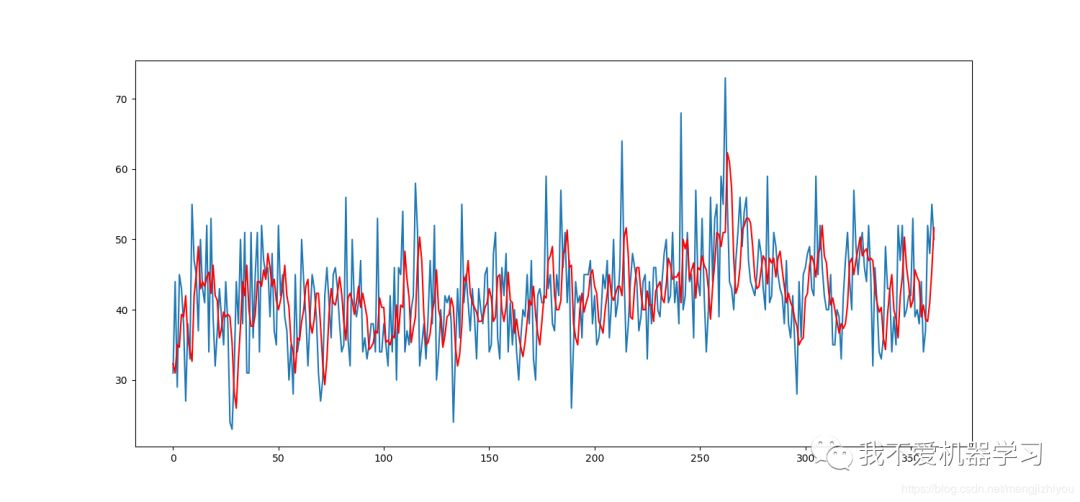以上就是移动平均的相关知识，希望对你有用。

建议阅读：

高考失利之后，属于我的大学本科四年

【AI蜗牛车出品】手把手AI项目、时空序列、时间序列、白话机器学习、pytorch修炼

公众号：AI蜗牛车

保持谦逊、保持自律、保持进步

个人微信
备注：昵称+学校/公司+方向
如果没有备注不拉群！
拉你进AI蜗牛车交流群


展开全文数据挖掘 人工智能 机器学习 python
• 该工具单个时间序列数据执行完整移动平均分析和完整趋势检测，并耦合时间序列之间的相关性执行完整相关分析。 “完整”是指系列中所有可能的子周期的分析。 它包括Mann-Kendall的tau A和tau B，Spearman的rho...matlab
• 差分整合移动平均自回归模型，又称整合移动平均自回归模型（移动也可称作滑动），是时间序列预测分析方法之
• 文章目录1.定义2.移动平均法、指数平滑法和季节模型1.移动平均法2.二次移动平均法及趋势移动平均法 1.定义 时间序列是按时间顺序排列的、随时间变化且相互关联的数据序列。...如果一个时间序列的概率分布与时间t无关

### 文章目录## 1.定义

时间序列是按时间顺序排列的、随时间变化且相互关联的数据序列。对时间序列进行观察研究，找寻它的发展规律，预测它将来的走势就是时间序列分析。

时间序列根据所研究的依据不同，可有不同的分类。

1. 按所研究的对象的多少分，有一元时间序列和多元时间序列.
2. 按时间的连续性可将时间序列分为离散时间序列和连续时间序列两种.
3. 按序列的统计特性分，有平稳时间序列和非平稳时间序列.如果一个时间序列的概率分布与时间t无关，则称该序列为严格的（狭义的）平稳时间序列。如果序列的一、二阶矩存在，而且对任意时刻t满足：
1. 均值为常数；
2. 协方差为时间间隔τ的函数

则该该序列为宽平稳时间序列，也叫广义平稳时间序列

对于这方面的内容，详情可以多参考概率论的随机过程部分-有相似之处。

1. 按时间序列的分布规律来分，有高斯型时间序列和非高斯型时间序列。

本章的主要内容是分析一元的时间序列分析。

## 2.移动平均法、指数平滑法和季节模型

### 1.移动平均法

移动平均法是常用的时间序列预测方法，由于其简单而具有很好的实用价值.

设观测序列为y1，···，yT，取移动平均的项数N<T.一次移动平均值计算公式为

M t ( 1 ) ( N ) = 1 N ( y t + y t − 1 + ⋅ ⋅ ⋅ + y t − N + 1 ) = 1 N ∑ i = 0 N − 1 y t − i M_t^{(1)}(N)=\frac{1}{N}(y_t+y_{t-1}+···+y_{t-N+1})=\frac{1}{N}\sum_{i=0}^{N-1}y_{t-i}

则有：

M t ( 1 ) ( N ) = 1 N ( y t − 1 + ⋅ ⋅ ⋅ + y t − N ) + 1 N ( y t − y t − N ) = M t − 1 ( 1 ) ( N ) + 1 N ( y t − y t − N ) M_t^{(1)}(N)=\frac{1}{N}(y_{t-1}+···+y_{t-N})+\frac{1}{N}(y_t-y_{t-N)}=M_{t-1}^{(1)}(N)+\frac{1}{N}(y_t-y_{t-N})

t+1期的预测值为 y t + 1 ^ = M t ( 1 ) ( N ) \hat{y_{t+1}}=M_t^{(1)}(N)

其预测标准误差为：

S = ∑ t = N + 1 T ( y t ^ − y t ) 2 T − N S=\sqrt{\frac{\sum_{t=N+1}^{T}(\hat{y_t}-y_t)^2}{T-N}}

如 果 将 y t + 1 ^ 作 为 t + 1 期 的 实 际 值 ， 那 么 就 可 以 用 y t + 1 ^ = M t ( 1 ) ( N ) 计 算 第 t + 2 期 预 测 值 y t + 2 ^ . 一 般 地 ， 也 可 响 应 地 求 得 以 后 各 期 的 预 测 值 。 但 由 于 越 远 时 期 的 预 测 ， 误 差 越 大 ， 因 此 ∗ ∗ 一 次 移 动 平 均 法 一 般 仅 应 用 于 一 个 时 期 后 的 预 测 值 （ 即 预 测 第 t + 1 期 ) ∗ ∗ 如果将\hat{y_{t+1}}作为t+1期的实际值，那么就可以用\hat{y_{t+1}}=M_t^{(1)}(N)计算第t+2期预测值\hat{y_{t+2}}.一般地，也可响应地求得以后各期的预测值。但由于越远时期的预测，误差越大，因此**一次移动平均法一般仅应用于一个时期后的预测值（即预测第t+1期)**

栗子：

汽车配件某年1~12年月份的化油器销售量（单位：只）统计数据见下表中第2行，试用一次移动平均法预测下一年1月份的销售量.

化油器销售量及一次移动平均法预测值表：

月份123456789101112预测
yi423358434445527429502480384427446
N=3405412469467461452469455430419
N=5437439452466473444444448

分别取N=3，N=5，按预测公式

y t + 1 ^ ( 3 ) = M t 1 ( 3 ) = y t + y t − 1 + y t − 2 3 , t = 3 , 4 , ⋅ ⋅ ⋅ , 12 \hat{y_{t+1}}(3)=M_t^{1}(3)=\frac{y_t+{y_{t-1}}+y_{t-2}}{3},t=3,4,···,12

y t + 1 ^ ( 5 ) = M t 1 ( 3 ) = y t + y t − 1 + y t − 2 + y t − 3 + y t − 4 3 , t = 5 , 6 , ⋅ ⋅ ⋅ , 12 \hat{y_{t+1}}(5)=M_t^{1}(3)=\frac{y_t+{y_{t-1}}+y_{t-2}+y_{t-3}+y_{t-4}}{3},t=5,6,···,12

计算3个月和5个月移动平均预测值，分别见上表第三行和第四行。N=3时，预测的标准误差为56.5752；N=5时，预测的标准误差为39.8159.

通过预测后，可以看到，实际数据波动较大，经移动平均后，随机波动明显减少，且N越大，波动也越小。同时，也可以看到，一次移动平均法的预测标准误差还是有些大，对于实际数据波动较大的序列，一般较少采用此法进行预测。

代码实现：

import numpy as np
y=np.array([423,358,434,445,527,429,426,502,480,384,427,446])
def MoveAverage(y,N):
Mt=['*']*N
for i in range(N+1,len(y)+2):
M=y[i-(N+1):i-1].mean()
Mt.append(M)
return Mt
yt3=MoveAverage(y,3)
s3=np.sqrt(((y[3:]-yt3[3:-1])**2).mean())
yt5=MoveAverage(y,5)
s5=np.sqrt(((y[5:]-yt5[5:-1])**2).mean())
print('N=3时,预测值：',yt3,'，预测的标准误差：',s3)
print('N=5时,预测值：',yt5,'，预测的标准误差：',s5)


简单移动平均使用的是等量加权策略，可以利用卷积，相应代码如下：

def sma(arr,n):

​	weights=np.ones(n)/n

​	return np.convolve(weights,arr)[n-1:-n+1]

import numpy as np
y=np.array([423,358,434,445,527,429,426,502,480,384,427,446])
n1=3; yt1=np.convolve(np.ones(n1)/n1,y)[n1-1:-n1+1] ##左开右闭 np.convolve(np.ones(n1)/n1,y,mode='valid')  同样适用-且更加具有普适性
s1=np.sqrt(((y[n1:]-yt1[:-1])**2).mean())
n2=5; yt2=np.convolve(np.ones(n2)/n2,y)[n2-1:-n2+1]
s2=np.sqrt(((y[n2:]-yt2[:-1])**2).mean())
print('N=3时,预测值：',yt1,'，预测的标准误差：',s1)
print('N=5时,预测值：',yt2,'，预测的标准误差：',s2)

np.convolve(a, v, mode='full'):
Returns the discrete, linear convolution of two one-dimensional sequences.

The convolution operator is often seen in signal processing, where it
models the effect of a linear time-invariant system on a signal _.  In
probability theory, the sum of two independent random variables is
distributed according to the convolution of their individual
distributions.

If v is longer than a, the arrays are swapped before computation.

Parameters
----------
a : (N,) array_like
First one-dimensional input array.
v : (M,) array_like
Second one-dimensional input array.
mode : {'full', 'valid', 'same'}, optional
'full':
By default, mode is 'full'.  This returns the convolution
at each point of overlap, with an output shape of (N+M-1,). At
the end-points of the convolution, the signals do not overlap
completely, and boundary effects may be seen.

'same':
Mode 'same' returns output of length max(M, N).  Boundary
effects are still visible.

'valid':
Mode 'valid' returns output of length
max(M, N) - min(M, N) + 1.  The convolution product is only given
for points where the signals overlap completely.  Values outside
the signal boundary have no effect.

Returns
-------
out : ndarray
Discrete, linear convolution of a and v.

--------
scipy.signal.fftconvolve : Convolve two arrays using the Fast Fourier
Transform.
scipy.linalg.toeplitz : Used to construct the convolution operator.
polymul : Polynomial multiplication. Same output as convolve, but also
accepts poly1d objects as input.

By default, mode is 'full'.  This returns the convolution
at each point of overlap, with an output shape of (N+M-1,). At
the end-points of the convolution, the signals do not overlap
completely, and boundary effects may be seen.
默认情况下，模式为“完整”。 这将返回卷积
在每个重叠点，总的输出形状为（N + M-1，）。 在
卷积的端点，信号不重叠
完全可以看到边界效应。
使用栗子：
Examples
--------
Note how the convolution operator flips the second array
before "sliding" the two across one another:

>>> np.convolve([1, 2, 3], [0, 1, 0.5])
array([0. , 1. , 2.5, 4. , 1.5])

Only return the middle values of the convolution.
Contains boundary effects, where zeros are taken
into account:

>>> np.convolve([1,2,3],[0,1,0.5], 'same')
array([1. ,  2.5,  4. ])

The two arrays are of the same length, so there
is only one position where they completely overlap:

>>> np.convolve([1,2,3],[0,1,0.5], 'valid')
array([2.5])

了解什么是卷积：
References
----------
..  Wikipedia, "Convolution",
https://en.wikipedia.org/wiki/Convolution

卷积公式可以描述为在时刻t处函数f（τ）的加权平均值，其中权重由g（–τ）给出，仅移动量t即可。随着t的变化，加权函数会强调输入函数的不同部分。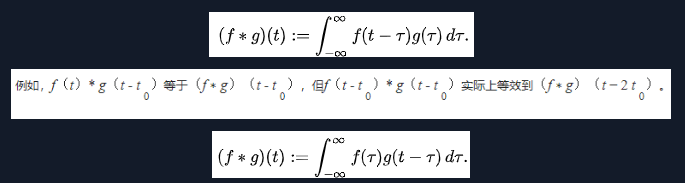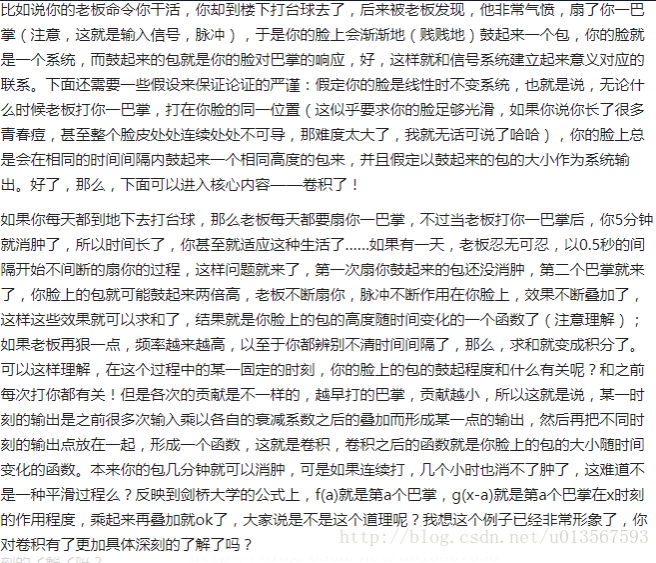离散序列的卷积求法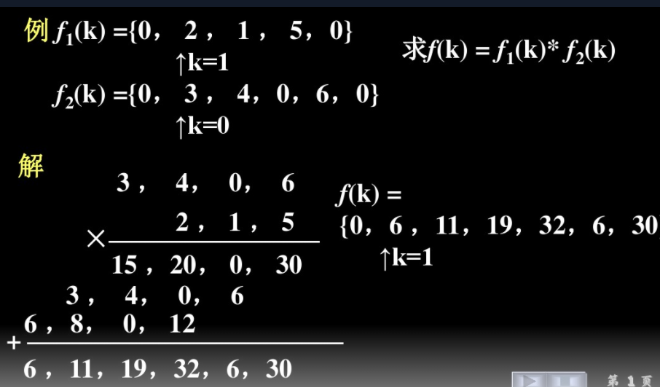卷积关键词：权重-时间-叠加性-离散还是连续

### 2.二次移动平均法及趋势移动平均法

当预测变量的基本趋势发生变化时，一次移动平均法不能迅速适应这种变化.

当时间序列的变化为线性趋势时，一次移动平均法的滞后偏差使预测值偏低，不能进行合理的趋势外推。

二次移动平均法-就是对一次移动平均数再进行二次移动平均，再以一次移动平均值和二次移动平均值为基础建立预测模型，计算预测值的方法。

二次移动平均值计算公式为：

M t ( 2 ) = 1 N ( M t ( 1 ) + ⋅ ⋅ ⋅ + M t − N + 1 ( 1 ) ) = M t − 1 ( 2 ) + 1 N ( M t ( 1 ) − M t − N ( 1 ) ) M_t^{(2)}=\frac{1}{N}(M_t^{(1)}+···+M_{t-N+1}^{(1)})=M_{t-1}^{(2)}+\frac{1}{N}(M_t^{(1)}-M_{t-N}^{(1)})

当预测目标的基本趋势是在某一水平上下波动时，可用一次移动平均方法建立预测模型。当预测目标的基本趋势与某一线性模型相吻合时，常用二次移动平均法.但序列同时存在线性趋势和周期波动时，可用趋势移动平均法建立预测模型:

y T + m ^ = a T + b T m , m = 1 , 2 , ⋅ ⋅ ⋅ , \hat{y_{T+m}}=a_T+b_T^{m},m=1,2,···,

其 中 a T = 2 M T ( 1 ) − M T ( 2 ) , b T = 2 N − 1 ( M T ( 1 ) − M T ( 2 ) ) . 其中a_T=2M_T^{(1)}-M_T^{(2)},b_T=\frac{2}{N-1}(M_T^{(1)}-M_T^{(2)}).

展开全文• 时间序列的其它博文系列： 时间序列模型 （）：模型概述 ...时间序列模型 （六）：平稳时间序列模型 ：自回归AR 、移动平均 MA 、ARMA 模型 时间序列模型 （七）： 时间序列建模的基本步骤 目录 移动平均法 ...
• 记录处理时间序列时需要用到的数据平滑方式 参考博客： 移动平均、指数平滑 三阶指数平滑 一阶指数平滑 1. 移动平均 import numpy as np import pandas as pd df = pd.DataFrame() df["data"] = np.random.rand(20...python 数据挖掘
• 之前介绍了时间序列的基本概念和性质，现在就正式介绍一些处理时间序列的模型方法，第一个移动平均法。 移动平均法很简单，就是用最近的数据预测未来短时间内的数据。有简单移动平均法，真的很简单，就是用最近的...指数平滑
• 电信设备-种基于移动平均时间序列模型的脑功能激活区检测方法.zip
•指数平均法
• 分析时间序列的方法构成数据分析的一个重要领域，即时间序列分析。 1．按所研究的对象的多少分，有一元时间序列和多元时间序列。 2．按时间的连续性可将时间序列分为离散时间序列和连续时间序列两种。 3．按序列的...
• 移动平均移动平均法是用组最近的实际数据来预测未来期或几期内目标值的常用方法。移动平均法适用于即期预测，当时间序列不会快速变化，且不存在季节性因素的时候，移动平均法能有效地消除预测中的...python 机器学习 人工智能 深度学习
• 近期学到《统计学》（贾俊平、何晓群、金勇进编著）的第13章——时间序列分析和预测，里面的“移动平均趋势剔除法”看得我一头雾水，尤其是第步的计算移动平均值，编者在书中一笔带过，不明所以的读者也只好脸懵...
• 季节性自回归综合移动平均（SARIMA）或季节性ARIMA是ARIMA的一个扩展，它明确支持具有季节性分量的单变量时间序列数据，它增加了三个新的超参数来指定序列季节性成分的自回归（AR）、差分（I）和移动平均（MA），...
• EXCEL时间序列分析-移动平均种预测方法是根据时间发展进行预测，简单来说就是时间序列预测。时间预测法的基本特点： (1)假设事物发展趋势会延伸到未来； (2)预测所依据的数据具有不规律性； (3)不考虑事物发展...
• 在本文中，它列出了MATLAB中可用的一些经典时间序列技术，您可以在探索机器学习方法之前先预测问题进行尝试。 它为您提供了每种方法的提示，以使您可以从一个有效的代码示例开始，并在哪里可以找到有关该方法的更...
• 时间序列与ARMA模型 自回归滑动平均模型（ARMA模型，Auto-Regression and Moving Average Model）是研究时间序列的重要方法，由自回归模型（AR模型）与滑动平均模型（MA模型）为基础“混合”而成，具有适用范围...ARMA
• 运用实际案例讲解如何使用Python编程的方式进行时间序列分析，...自回归移动平均（ARMA）建模方法自回归差分移动平均（ARiMA）建模方法时间序列分解方法（趋势与季节性分析）授课内容简洁明了，突出重点，强调实用性。python 大数据
• 时间序列的其它博文系列： 时间序列模型 （）：模型...时间序列模型 （六）：平稳时间序列模型 ：自回归AR 、移动平均 MA 、ARMA 模型 时间序列模型 （七）： 时间序列建模的基本步骤 这里的平稳是指宽平稳，...
• 时间序列（从现在起称为TS）被认为是数据科学领域中鲜为人知的技能之。 使用python创建时间序列预测 我们使用以下步骤： 时间序列是什么 加载和处理时间序列 如何检验时间序列的平稳性？ 如何使时间序列平稳...Python R ARIMA
• 了解ARIMA模型就要先了解数据的平稳性，样本数据获得的时间序列，在未来一段时间沿着一个“趋势”发展下去，只有具有平稳性的数据才可以做预测 平稳性的数据均值和方差不发生明显变化 方差越大数据的波动越大 ...机器学习
• ## 移动平均法详解

千次阅读 2020-12-07 13:10:02
什么是移动平均法?移动平均法是用组最近的实际数据值来预测未来期或几期内公司产品的需求量、公司产能等的种常用方法。...移动平均法是种简单平滑预测技术，它的基本思想是：根据时间序列...
• 在研究qqq阶移动平均过程之前，我们先以一阶移动平均过程开刀，即MA(1)MA(1)MA(1)序列, 一阶移动平均模型表达式为： Yt=et−θet−1(1) Y_t=e_t-\theta e_{t-1}\tag{1} Yt​=et​−θet−1​(1) 其...
• 原文： ...amp;variant=zh-cn#_note-0 移动平均（英语：moving average，MA），又称“移动平均线”简称均线，是技术分析中种分析时间序列数据的工具。最常见的是利用股价、回报或交易量等变数计算出移动平均。......